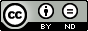### On New Solutions of System of Shallow Water Wave Equations

#### Abstract

Obtaining analytical solutions of nonlinear differential equations and nonlinear systems of partial and ordinary differential equations is an important topic in various fields of Mathematics. Many techniques are available in the literature. In this note, the enhanced modified simple equation method (EMSEM) is applied to system of shallow water wave equations.

#### Keywords

Nonlinear system of differential equation; analytical solutions; EMSEM;shallow water wave equations

PDF

#### References

J. H. He and X. H. Wu, “Exp-function method for nonlinear wave equations,” Chaos, Solitons & Fractals, vol. 30, no. 3, pp. 700-708, 2006.

R. Sakthivel, and C. Chun, “New Soliton Solutions of Chafee-Infante Equations using the Exp-Function Method,” Z. Naturforsch, 65a, pp. 197-202, 2010.

M. A. Abdou, “The extended Tanh method and its applications for solving nonlinear physical models,” Applied Mathematics and Computation, vol. 190, no. 1, pp. 988-996, 2007.

E. Fan, “Extended tanh-function method and its applications to nonlinear equations,” Physics Letters A, vol. 277, no. 4-5, pp. 212-218, 2000.

M. L. Wang, “Solitary wave solutions for variant Boussinesq equations,” Physics Letters A, vol. 199, no. 3-4, pp. 169-172, 1995.

E. M. E. Zayed, H. A. Zedan, and K. A. Gepreel, “On the solitary wave solutions for nonlinear Hirota-Satsuma coupled KdV of equations,” Chaos, Solitons & Fractals, vol. 22, no. 2, pp. 285-303,2004.

M. Wang, X. Li, and J. Zhang, “The (G’/G)-expansion method and travelling wave solutions of nonlinear evolution equations in mathematical physics, ” Physics Letters A, vol. 372, no. 4, pp. 417-423, 2008.

E. Aksoy, A. Bekir, “Exact solutions of shallow water wave equations by using the (G’/G)-expansion method,” waves in random and complex media, 22 (3), pp. 1-15, 2012.

M. R. Miura, Backlund Transformation, Springer, Berlin, Germany, 1978.

D. Lu and Q. Shi, “New Jacobi elliptic functions solutions for the combined KdV-MKdV equation,” International Journal of Nonlinear science, vol. 10, no. 3, pp. 320-325, 2010.

A. J. M. Jawad, M. D. Petkovic’, and A. Biswas, “Modified simple equation method for nonlinear evolution equations,” Applied Mathematics and Computation, vol. 217, no. 2, pp. 869-877, 2010.

E. M. E. Zayed and S. A. H. Ibrahim, “Exact solutions of nonlinear evolution equations in mathematical physics using the modified simple equation method,” Chinese Physics Letters, vol. 29, no. 6, Article ID 060201, 2012.

C. Zhang and Z. Zhang, “Application of the enhanced modified simple equation method for Burger-Fisher and modified Volterra equations,” Advances in Difference Equations, 2017

DOI: https://doi.org/10.24203/ajas.v7i6.5989

### Refbacks

• There are currently no refbacks.